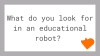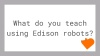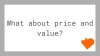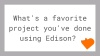GLOBAL SITE AUSTRALIAN SITE
Select Page

# LESSON 3: Robot Movement – Turning

Sequential programing and basic geometry – Students learn how the robot responds to time and geometry and how they can achieve driving control of the robot.

In this lesson students will use the basic control drive icons to turn the robot at different angles (90°, 180° and 270°) and then using learning from lesson 2 create a driving sequence.

## Program 1 – Right turn (Worksheet 3.1)

Using either Activity sheet 3.1 or coloured tape on a desk, instruct the students to write a program that turns the robot right 90 degrees.

## Program 2 – Left turn (Worksheet 3.2)

Using either worksheet 3.1 or coloured tape on a desk, instruct the students to write a program that turns the robot left 180 degrees.

## Program 3 – Right and then left turn (Worksheet 3.3)

Using either worksheet 3.1 or coloured tape on a desk, instruct the students to write a program that turns the robot 90 degrees right then 270 (90 + 180) degrees left.

## Program 4 – Mini maze (Worksheet 3.4)

Using either worksheet 3.2 or coloured tape on a desk, instruct the students to write a program that can navigate a mini maze. Students will use the learning from lesson 2 and 3 to complete this challenge.

Each worksheet asks students to describe what the robot is doing and why. A typical answer may look like:

The robot turns because the program has a drive icon that states spin left. It turns 90 degrees because the time in the event wait icon is 0.35 seconds and this is how long it takes the robot to turn that far.

• ### Keep up with Edison

• This field is for validation purposes and should be left unchanged.